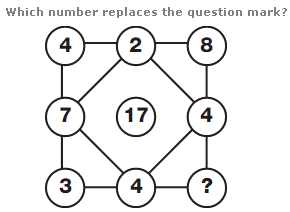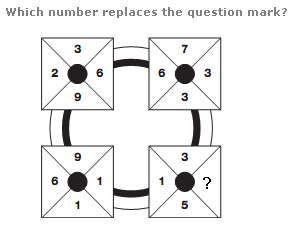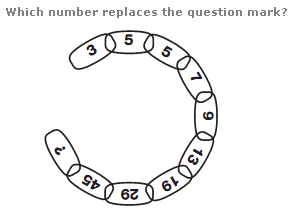# Puzzles - Number puzzles

### Exercise :: Number puzzlesAnswer : 2 Explanation : The sum of the 4 digits at the corners of the diagram is 17, as is the sum of the 4 digits in the middle of each side of the diagram.Answer : 9 Explanation : Starting with the top left square, and moving clockwise around the other 3, the sum of the digits in each square follows the sequence 20, 19, 18, 17.Answer : 71 Explanation : As you move clockwise around the diagram, add the previous 2 numbers together and subtract 3 to give the next number in the chain.